GALILEO

## Galileo EPD Handbook

### Chapter 1. Instrument Summary

Charged Particle Response of Magnetic Deflection System for Galileo Jupiter Orbiter (draft)

Yue Wu and T. P. Armstrong, Dept. of Physics and Astronomy, University of Kansas, Lawrence, KS 66045

Note: The final version of this paper was published in Nuclear Instruments and Methods in Physics Research, A265, 561, 1988.

Abstract. A simulation of a magnetic spectrometer system and the study of its instrumental response are presented here. An analytic, three-dimensional expression for the inhomogeneous and fringing magnetic field of the system is obtained. A three-dimensional, time-reversed trajectory calculation of electrons and protons inside the system is performed. A clean electron-ion separation and electron analysis are achieved. The geometric factors of the electron and proton detectors for different energies and the particles' angular distributions are determined. It is found that the geometric factors of two electron detectors, E and F, have an appreciable variation for different energies with a maximum at 70 and 500 keV, respectively, whereas the proton detector has monotonic increasing geometric factors as the energy increases.

Acknowledgements. This work was supported by NASA through the Galileo program. The principal investigator for the EPD experiment is D. J. Williams of the Johns Hopkins University Applied Physics Laboratory.

Figures: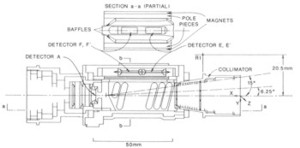Figure 1. Cross section of LEMMS assembly. R1 is the reference point for the magnetic field, and it is also the origin of the coordinates used in this study. E is the lower energy electron detector, F the higher energy electron detector, and A is the proton detector.Figure 2. Left: Cross section of the magnetic and pole pieces. Right: aperture area display.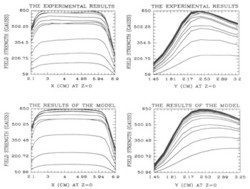Figure 3. Comparison between measurements and results of the model. Each curve corresponds to a different specific value of y (left) and x (right).Figure 4. Projection of the magnetic field lines in the y-z plane at x=4.32 cm (left panel) and in the x-z plane at y=4.18 cm (right panel). The maximum field strength at z=0 is 650 G.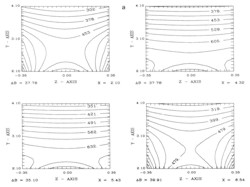Figure 5a. Contour plots of the magnitude of the magnetic field.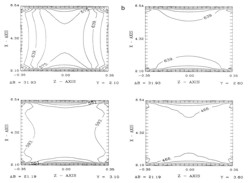Figure 5b. Contour plots of the magnitude of the magnetic field.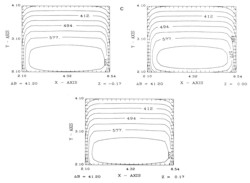Figure 5c. Contour plots of the magnitude of the magnetic field.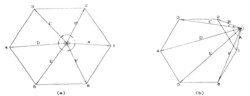Figure 6. (a) The directions of A x B, B x C, C x D, D x E, E x F, and F x A remain unchanged; therefore the intersection point lies inside the polygon. (b) The directions of A x B and B x C are opposite; therefore the intersection point lies outside the polygon.Figure 7. A segment of electron trajectory intersects with a detector.  ΔA is the sample area on the detector and θand φ are the polar and azimuthal angles, respectively.Figure 8. The mapping of angular distributions at detector E and apertures.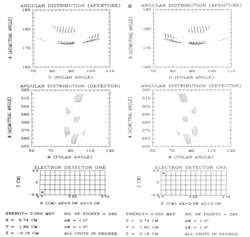Figure 9a. Angular distributions of 50 keV electrons for two symmetric sample points with respect to the middle plane.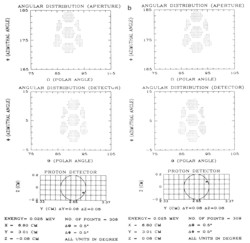Figure 9b. Angular distributions of 25 keV protons for two symmetric sample points with respect to the middle plane.Figure 9c. Comparison of the angular distributions for 50 (top), 80 (center), and 100 keV (bottom) electrons emanating from the center of detector E with x=3.22 cm and y=1.82 cm.Figure 10. Geometric factors of detectors of the Galileo LEMMS sensor.